### Simple Linear Regression from ScratchIn this example, we'll learn how to do simple linear regression.

In linear regression we have an input data $X$ and we'll assume that our output data $Y$ depends on $X$ via some linear relationship. So to give an example you can imagine $X$ being your monthly income and $Y$ being the amount you can save each month. Let's say you can save 20% of your income.
The amount you can save is given by the equation: $Y = X * 0.2$. Here your savings depend on your income linearly (times 0.2).
Normally the goal of linear regression is to find such relationships in the data. So assume that we survey 100 people, ask for their monthly income and monthly savings and we'll try to figure out the relationship between people's income and outcome.
Just as with many other statistical methods, the problem boils down to optimisation. We come up with a guess for the relationship, see how badly we guessed and adjust our guess to make better guesses. This means that we're optimising our error function, which is usually the distance between the predicted $Y$s and the observed $Y$s.
In this case the problem is so simple that there is a very simple closed form solution that we can apply. Let's call this optimal parameter $β$, then the ordinary least squares estimator is equal to:
$β = (X^T * X)^{-1} * X^T * Y$
There are loads of resources on the derivation of this, so I won't repeat this here.
You can look here or here.
Let's see how we can implement this in Julia.
First, let's set up our input data $X$:
julia> X = 1000 .+ (rand(100) - 0.5) * 100
100-element Array{Float64,1}:
952.315
969.791
1002.52
1007.6
1005.87
1031.43
953.516
1036.9
980.244
976.039
⋮
1007.23
1011.37
958.018
978.313
957.968
1024.01
965.105
1047.75
951.604

julia> using Plots

julia> pyplot(size = (600, 600))
Plots.PyPlotBackend()

julia> histogram(X)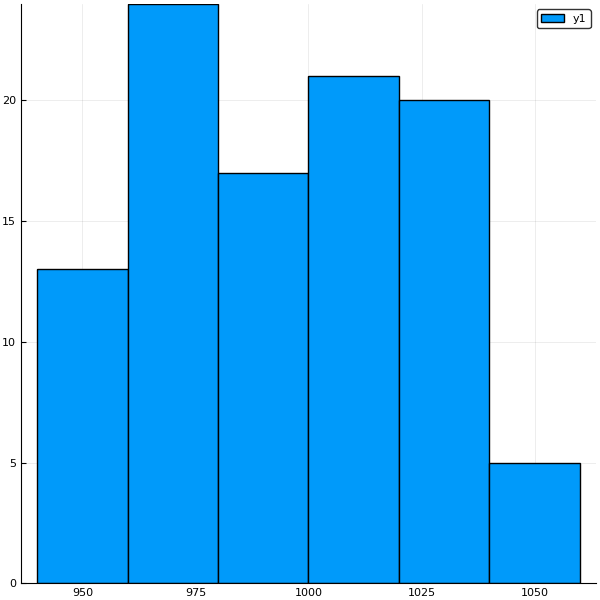This represents the income of the respondents. We can see that the average income is uniformly distributed around £1000.
Let's create $Y$:
julia> Y = X .* 0.2 .+ randn(size(X,1));

I've added some random noise to the $Y$ variable, as not everyone is saving exactly 20% of their income. You can also think of this as measurement error.
Now let's plot the 2 variables together:
julia> scatter(X, Y, marker = (5))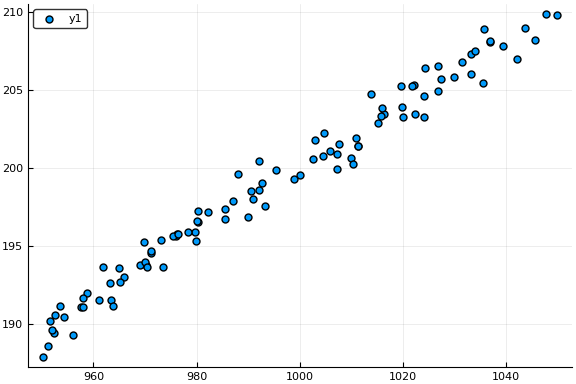We can definitely see that there is clear linear relationship between $X$ and $Y$. As $X$ increases, $Y$ increases too. (This is of course by design here). Let's hope that our algorithm can pick this up.
Using the closed form solution above, we create a function to estimate $β$:
julia> function lm_closed(X, Y)
# ': denotes the transpose of the matrix, while inv gives us the inverse
β = inv(X' * X) * X' * Y
return(β)
end
lm_closed (generic function with 1 method)

julia> β_new = lm_closed(X, Y)
0.2000155230286191

So it looks like it did find the correct 0.2 value. It is not exactly 0.2 as there is some noise in the data.
If we add this relationship to the scatter plot above as line, we get the following:
julia> plot!(x -> x * β_new, line = (3))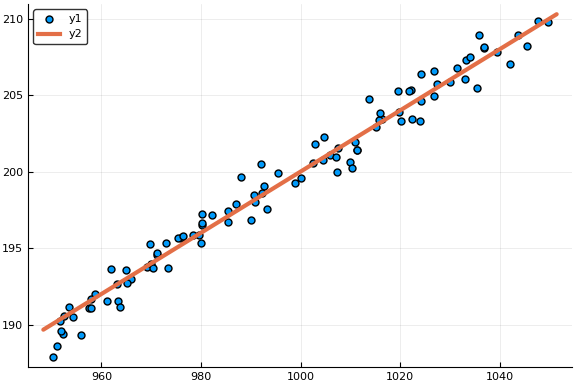Pretty cool!
But what if everyone gets £100 grant every month that they must save? Then people would save 20% of their income and an extra £100 regardless of their income. Can our code handle that?
julia> Y2 = X .* 0.2 .+ randn(size(X,1)) .+ 100;

julia> β_grant = lm_closed(X, Y2)
0.30044461036726283

Hmmm... Our estimator now thinks that everyone is saving 30% of their income. This is true for people earning £1000 (£100 + £200), but it's not true for people earning £3000. With the current $β_{grant}$, we'd estimate their savings at £3000 * 0.3 = £900, whereas in reality, they'd only save £3000 * 0.2 + £100 = £700. That's a massive £200 overestimation!
This is happening as our model is not flexible enough to model such situations. It is only able to model simple multiplicative relationships, but not the additive £100. We call that term the bias and it's fairly simple to introduce it to our model. All we need to do is add an extra column of 1s to our X and we're done.
julia> function lm_closed_bias(X, Y)
n_obs = size(X, 1)

# here ones() gives a vector of length n_obs filled with floating point 1.0s
# we concatonate this to X horizontally with hcat.
# I.e. stick them together. The first column is 1s and the
# rest are the columns of X
X = hcat(ones(Float64, n_obs), X)

β = inv(X' * X) * X' * Y
return(β)
end
lm_closed_bias (generic function with 1 method)

julia> β_bias = lm_closed_bias(X, Y2)
2-element Array{Float64,1}:
103.903
0.196134

Now $β$ has length 2. The first term represents the bias term, which is close to £100 and the second the multiplicative term, close to 20%. Yay! We managed to make our model more flexible to be able to model such "complex" relationships.
Let's compare the bias and non-bias models against each other.
julia> pyplot(size= (600, 600))
Plots.PyPlotBackend()

julia> scatter(X, Y2, marker = (5))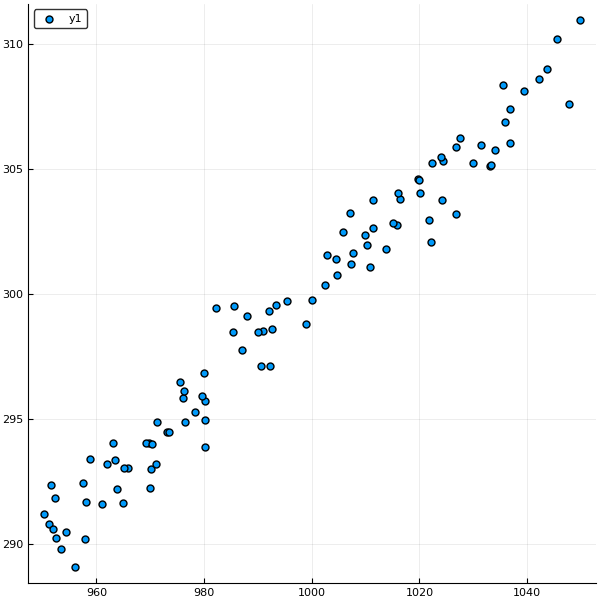julia> plot!(x -> x * β_grant, line = ("red", 3))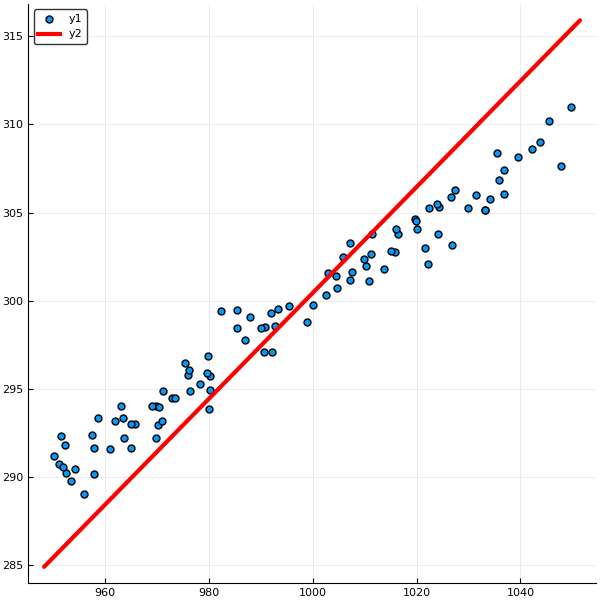julia> plot!(x -> β_bias + x * β_bias, line = ("green", 3))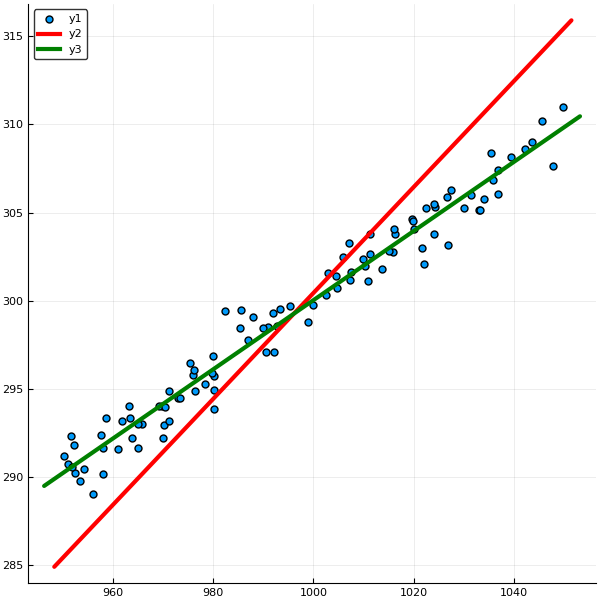It's clear that the green line is a lot better fit than the red one.
Now this has been fun and all and I hope that this post helped you build some intuition on how ordinary least squares (OLS) works with some simple examples. In practice, it's usually better to use packages built for this sort of work. Here's how you can build the same regressors using the GLM.jl package.
julia> using GLM, DataFrames

julia> df = DataFrame(X = X, Y = Y2)
100×2 DataFrames.DataFrame
│ Row │ X       │ Y       │
├─────┼─────────┼─────────┤
│ 1   │ 952.315 │ 291.806 │
│ 2   │ 969.791 │ 294.043 │
│ 3   │ 1002.52 │ 300.349 │
│ 4   │ 1007.6  │ 301.651 │
│ 5   │ 1005.87 │ 302.471 │
│ 6   │ 1031.43 │ 305.973 │
│ 7   │ 953.516 │ 289.795 │
│ 8   │ 1036.9  │ 307.392 │
⋮
│ 92  │ 1007.23 │ 301.209 │
│ 93  │ 1011.37 │ 303.769 │
│ 94  │ 958.018 │ 291.684 │
│ 95  │ 978.313 │ 295.282 │
│ 96  │ 957.968 │ 290.198 │
│ 97  │ 1024.01 │ 305.478 │
│ 98  │ 965.105 │ 293.033 │
│ 99  │ 1047.75 │ 307.63  │
│ 100 │ 951.604 │ 292.348 │

julia> fit_lm = glm(@formula(Y ~ X),
df,
Normal())

Formula: Y ~ 1 + X

Coefficients:
Estimate  Std.Error z value Pr(>|z|)
(Intercept)   103.903     3.6696 28.3145   <1e-99
X            0.196134 0.00368549 53.2179   <1e-99

You can extract the coefficients $β$ from the models using the coef() function.
julia> coef(fit_lm)
2-element Array{Float64,1}:
103.903
0.196134

julia> plot!(x -> [1, x]' * coef(fit_lm), line = ("purple", :dot, 8))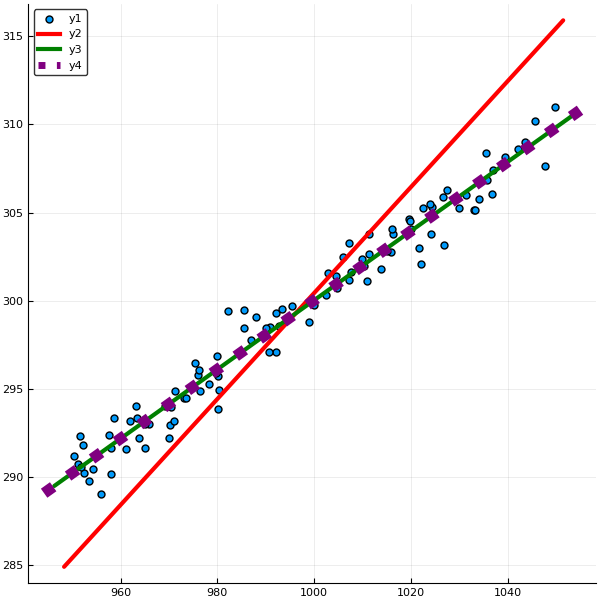Good to know that our solution was spot on (purple dots overlaying our green line)! Thanks for reading trough this post. If you have any questions/suggestions/requests feel free to leave a comment!